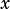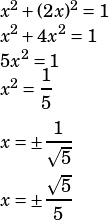in the xy plane, point (x,y) lies on the circle with equation x^2+y^2=1 and on the line with the equation y=2x what is the value of xy?
It sat math subject test level 1

Let’s get this by substitution. We know, so let’s plug that into the circle equation and solve for.This makes sense.is a line that goes through the origin, andis a circle centered on the origin. So we know we’ll either have a negativeand a negative, or we’ll have a positiveand a positive.

So let’s just plug the positive-value back into.Now all that’s left is multiplication. The question asks for the value of.